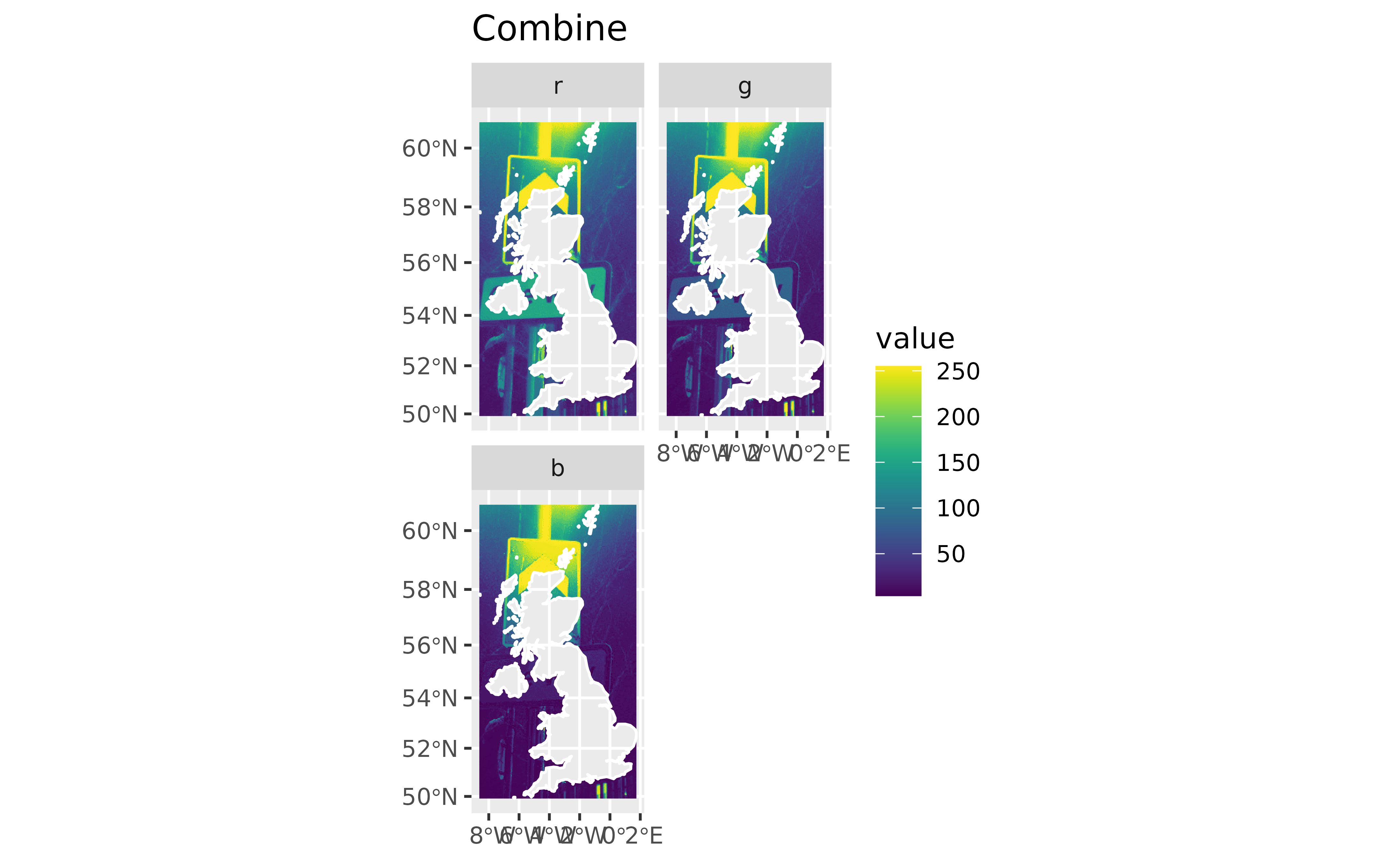Geotags an image based on the coordinates of a given spatial object.

## Usage

rasterpic_img(
x,
img,
halign = 0.5,
valign = 0.5,
expand = 0,
crop = FALSE,
inverse = FALSE,
crs
)

## Arguments

x

It could be

• A sf, sfc, sfg or bounding box (see sf::st_bbox()) object (sf package).

• A SpatRaster, SpatVector or SpatExtent object (terra package).

• A numeric vector of length 4 with the extent to be used for geotagging ( i.e. c(xmin, ymin, xmax, ymax)).

img

An image to be geotagged. It can be a local file or an online file (e.g. "https://i.imgur.com/6yHmlwT.jpeg"). The following image extensions are accepted:

• png

• jpeg/jpg

• tiff/tif

halign

Horizontal alignment of img with respect to the x object. It should be a value between 0 (x is aligned on the left edge of the raster) and 1 (x is on the right edge of the raster).

valign

Vertical alignment of img with respect to the x object. It should be a value between 0 (x is aligned on the bottom edge of the raster) and 1 (x is on the top edge of the raster).

expand

An expansion factor of the bounding box of x. 0 means that no expansion is added, 1 means that the bounding box is expanded to double the original size.

crop

Logical. Should the raster be cropped to the (expanded) bounding box of x?

Logical. Should the raster be masked to x? See terra::mask() for details. This option is only valid if x is a sf/sfc object.

inverse

Logical. It affects only if mask = TRUE. If TRUE, areas on the raster that do not overlap with x are masked.

crs

Character string describing a coordinate reference system. This parameter would only affect if x does not present a Coordinate Reference System (e.g. when x is a SpatExtent, sfg bbox or a vector of coordinates). See Details

## Value

A SpatRaster object.

## Details

The function preserves the Coordinate Reference System of the x object. For optimal results do not use geographic coordinates (longitude/latitude).

crs can be in a WKT format, as a "authority:number" code such as "EPSG:4326", or a PROJ-string format such as "+proj=utm +zone=12". It can be also retrieved as sf::st_crs(25830)$wkt. See value and Notes on terra::crs(). ## See also sf::st_crs(), sf::st_bbox(), terra::crs(). ## Examples # \donttest{ library(sf) #> Linking to GEOS 3.9.1, GDAL 3.3.2, PROJ 7.2.1; sf_use_s2() is TRUE library(terra) x_path <- system.file("gpkg/UK.gpkg", package = "rasterpic") x <- st_read(x_path, quiet = TRUE) img <- system.file("img/vertical.png", package = "rasterpic") # Default config ex1 <- rasterpic_img(x, img) class(ex1) #>  "SpatRaster" #> attr(,"package") #>  "terra" plotRGB(ex1) plot(x$geom,
col = NA,
border = "white",
lwd = 2
)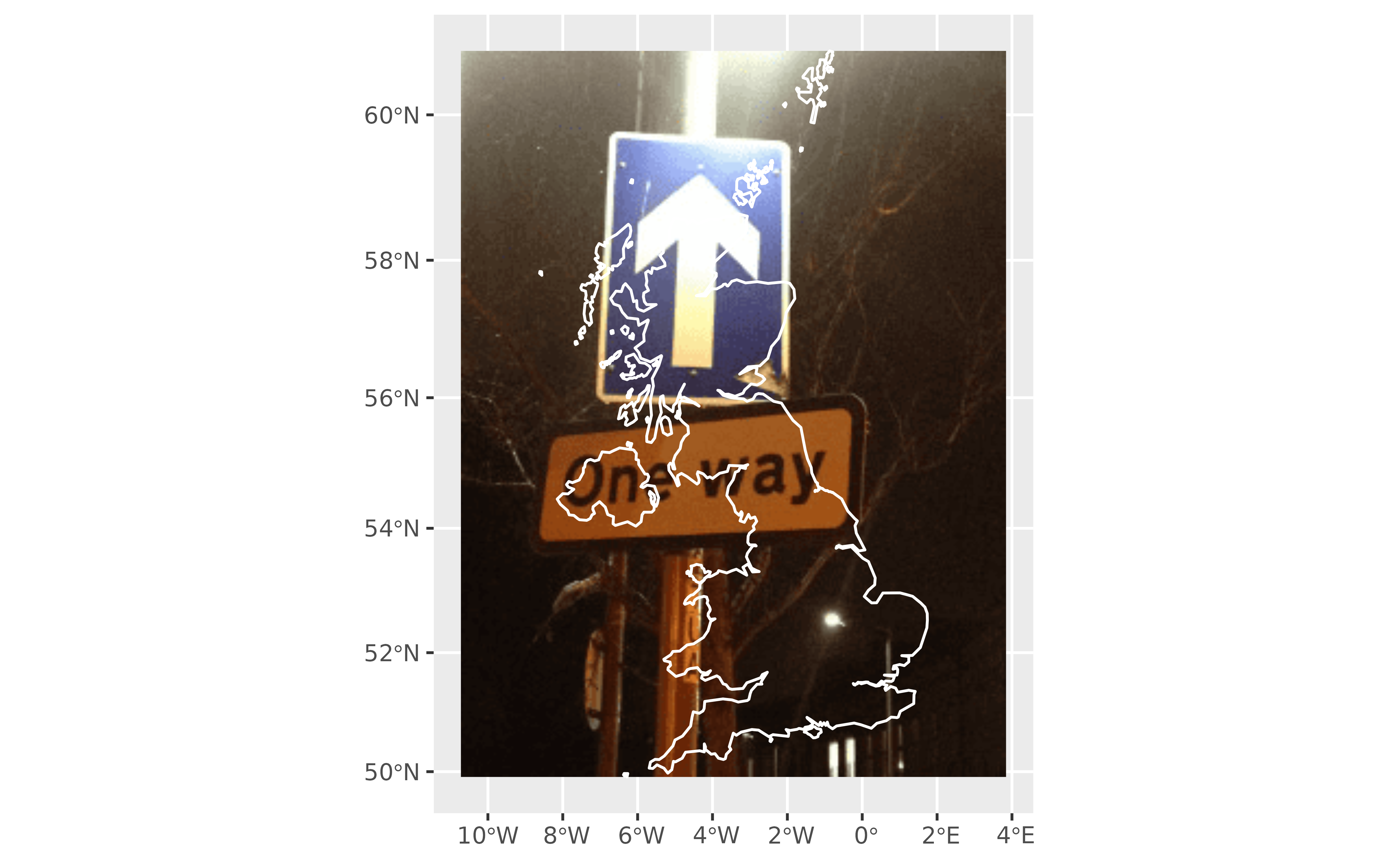# Expand
ex2 <- rasterpic_img(x,
img,
expand = 0.5
)

plotRGB(ex2)
plot(xgeom, add = TRUE, col = NA, border = "white", lwd = 2 )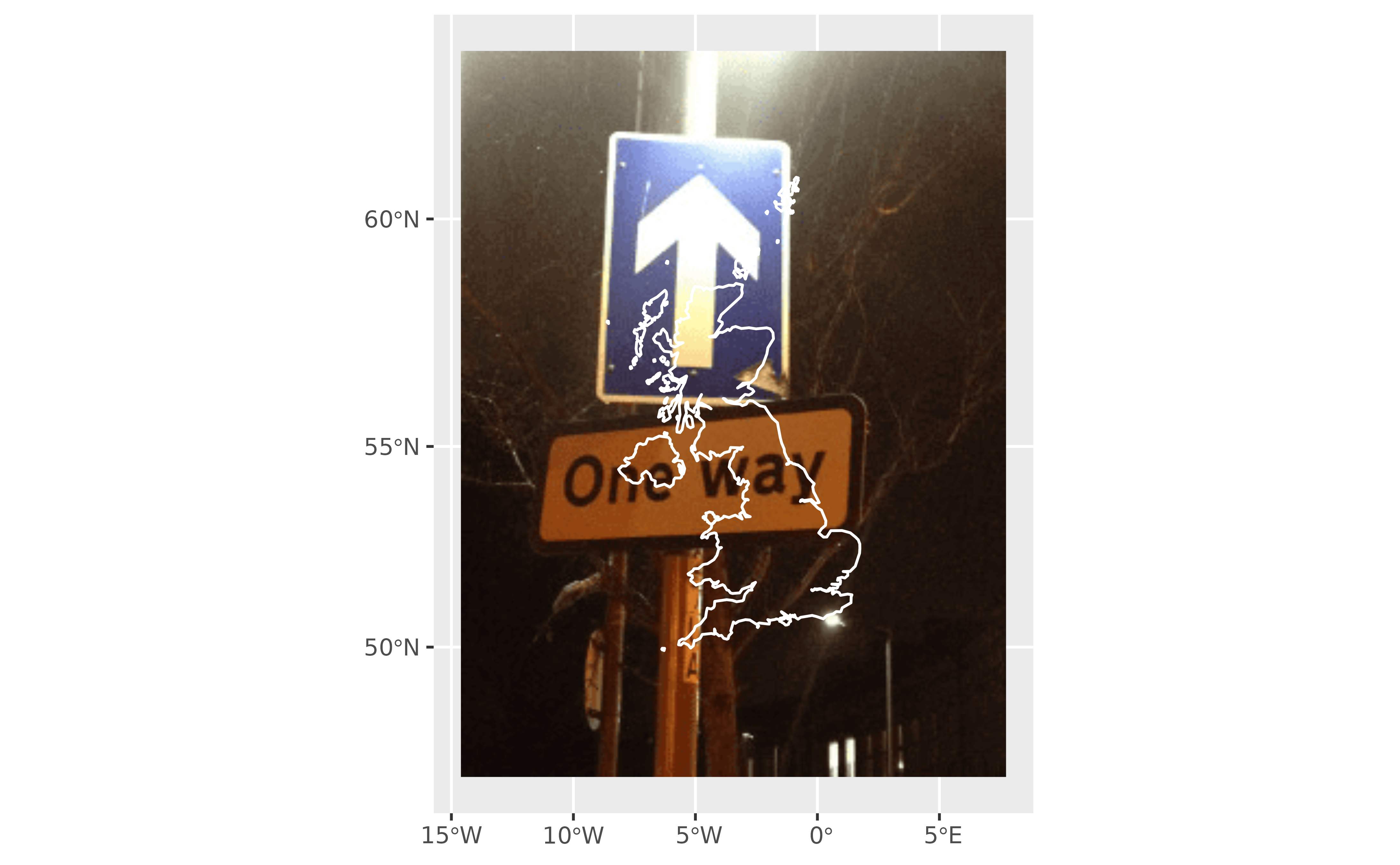# Align ex3 <- rasterpic_img(x, img, halign = 0 ) plotRGB(ex3) plot(xgeom,
col = NA,
border = "white",
lwd = 2
)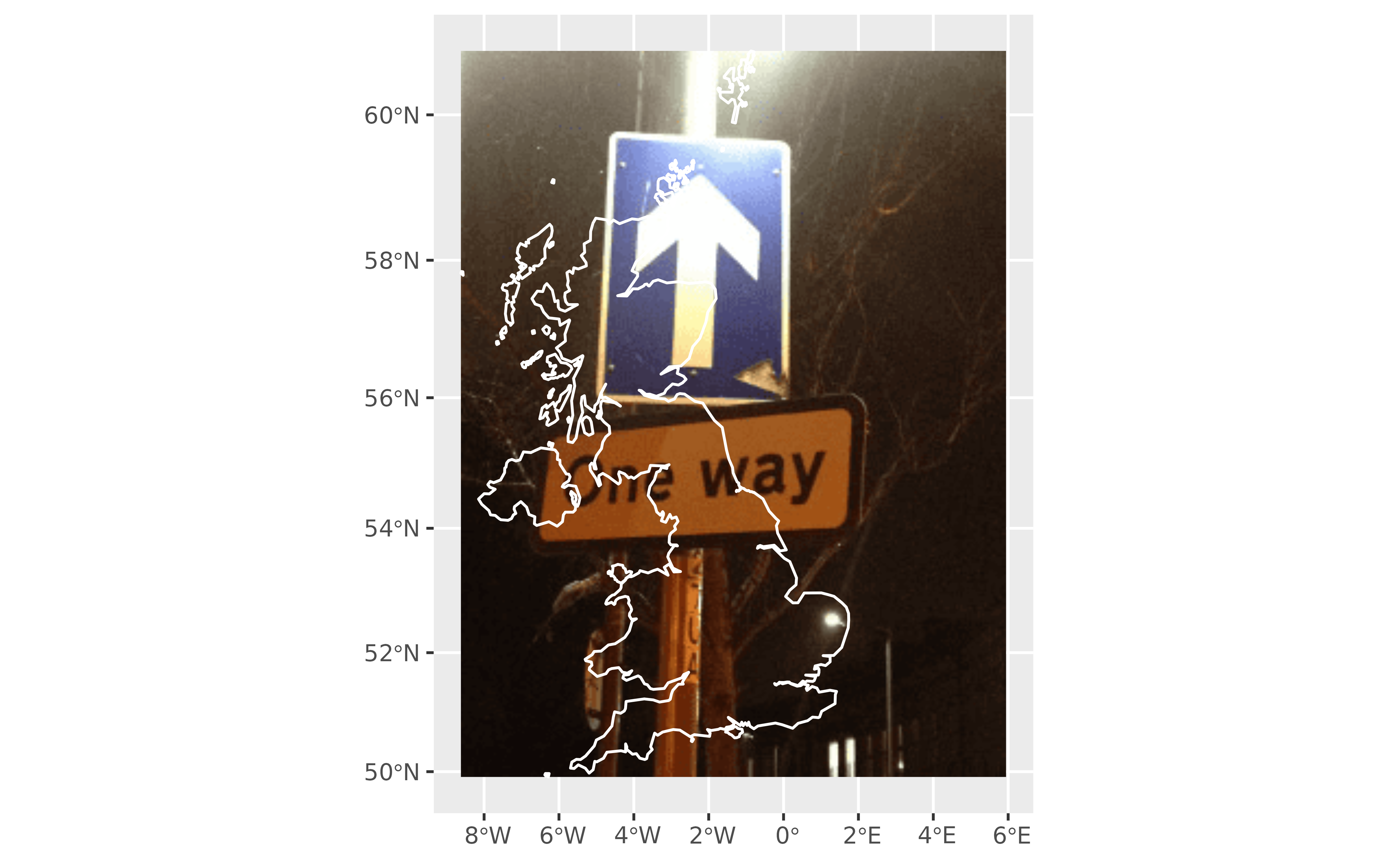# Crop
ex4 <- rasterpic_img(x,
img,
crop = TRUE
)

plotRGB(ex4)
plot(x$geom, add = TRUE, col = NA, border = "white", lwd = 2 )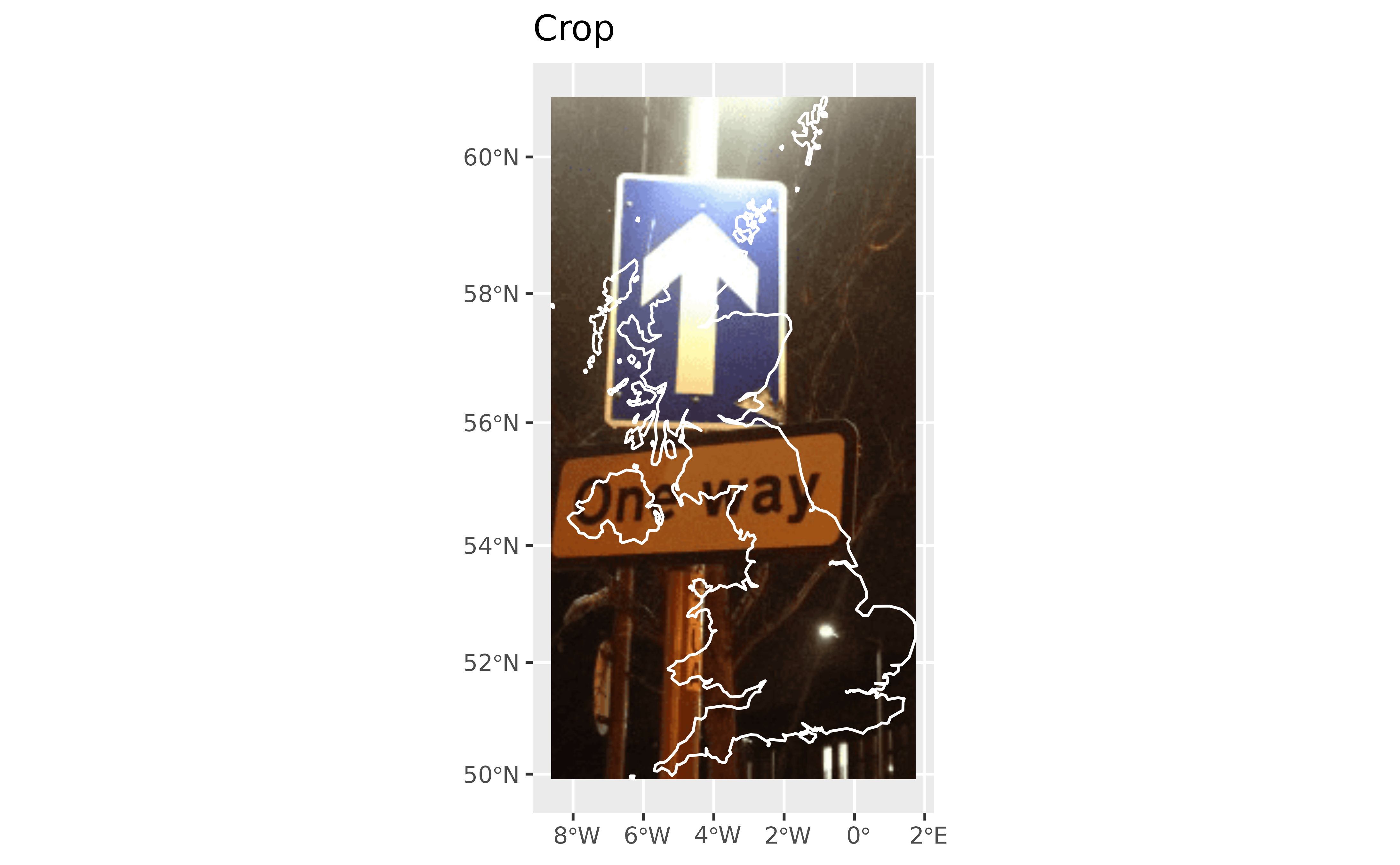# Mask ex5 <- rasterpic_img(x, img, mask = TRUE ) plotRGB(ex5) plot(x$geom,
col = NA,
border = "white",
lwd = 2
)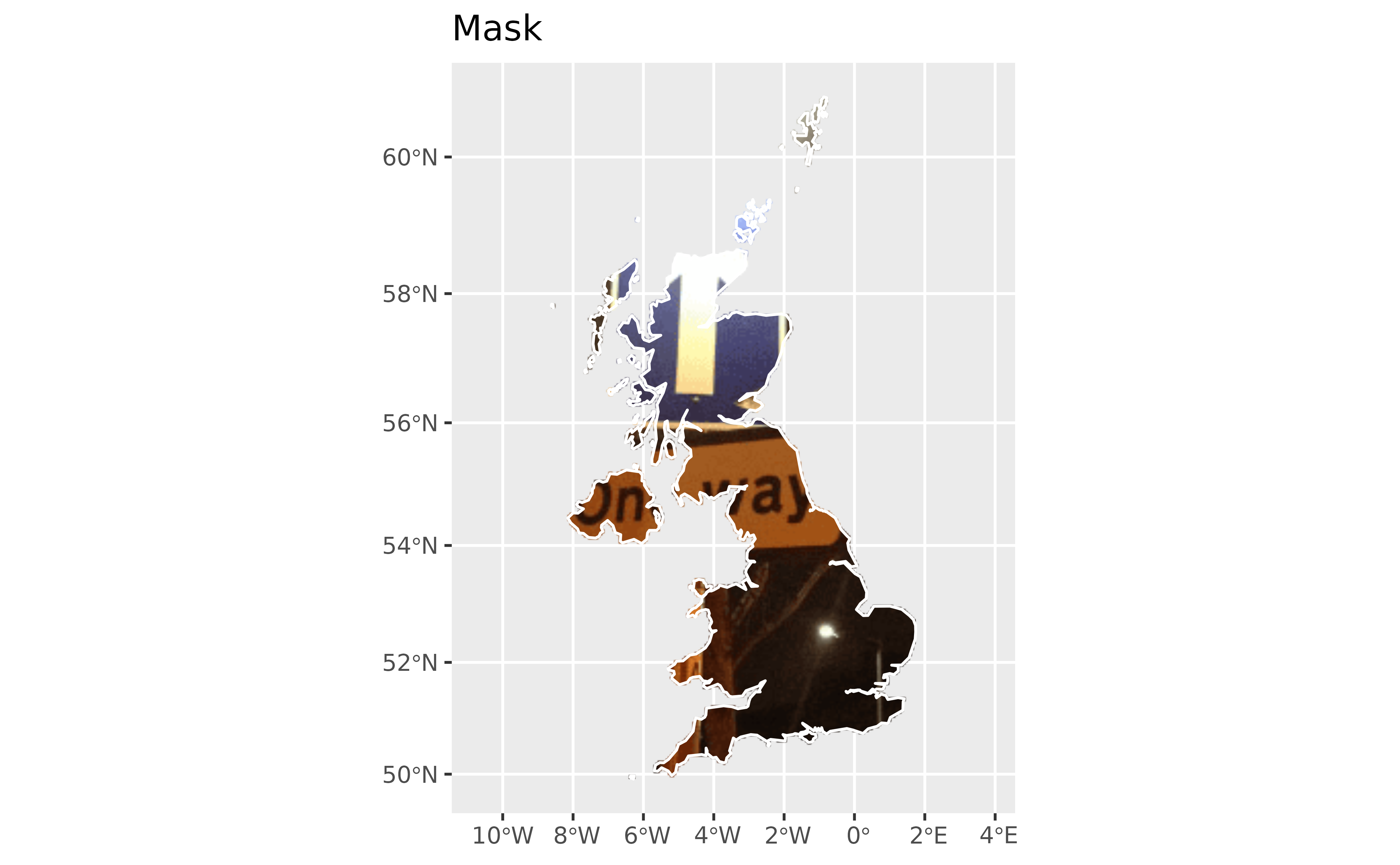ex6 <- rasterpic_img(x,
img,
plot(x$geom, add = TRUE, col = NA, border = "white", lwd = 2 )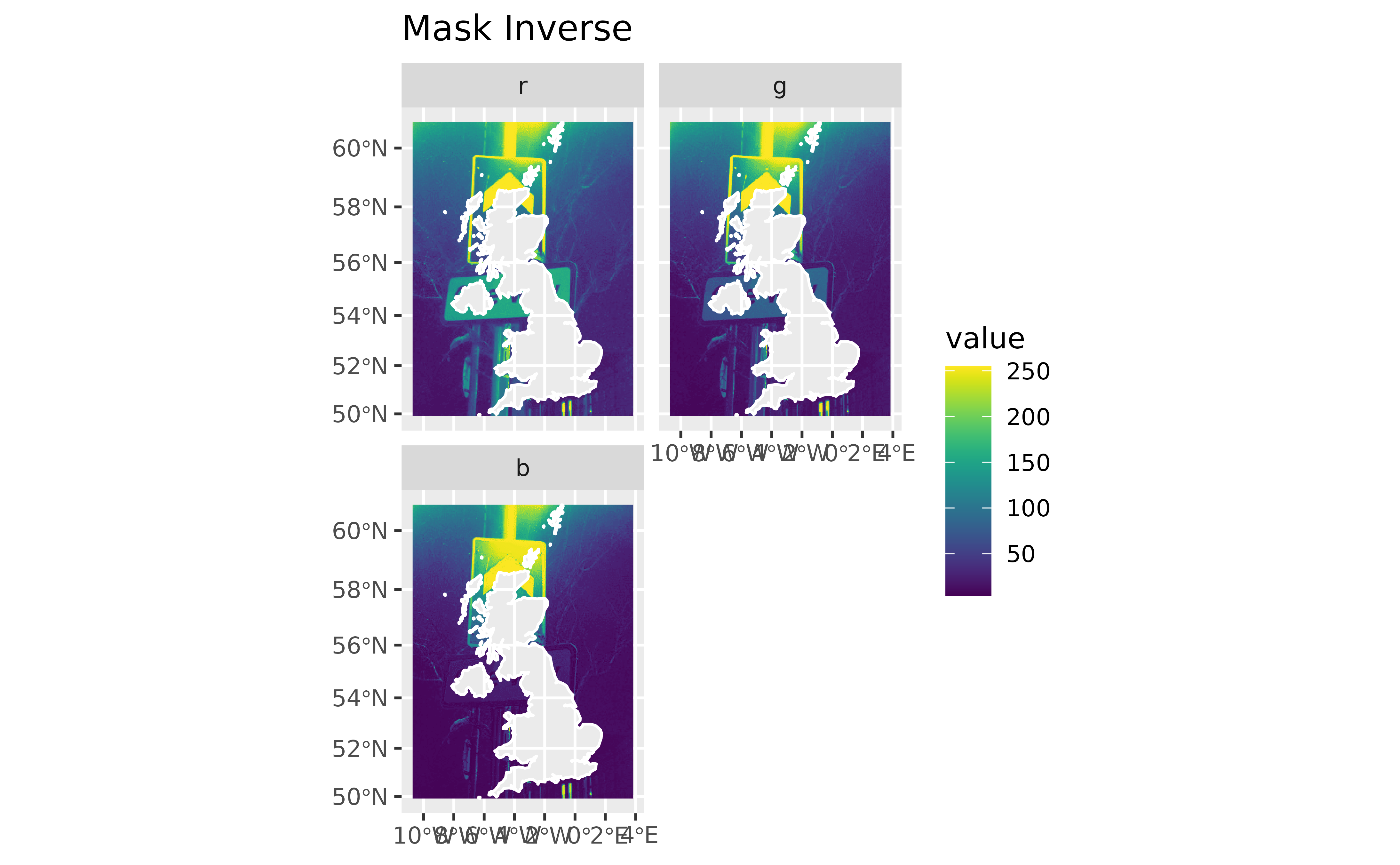# Combine Mask inverse and crop ex7 <- rasterpic_img(x, img, crop = TRUE, mask = TRUE, inverse = TRUE ) plotRGB(ex7) plot(x$geom,